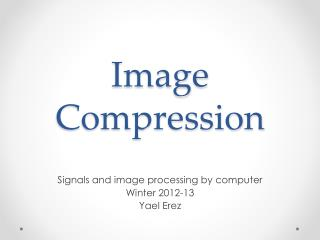DownloadDownload PresentationImage Compression

# Image Compression

Télécharger la présentation## Image Compression

- - - - - - - - - - - - - - - - - - - - - - - - - - - E N D - - - - - - - - - - - - - - - - - - - - - - - - - - -
##### Presentation Transcript

1. Image Compression Signals and image processing by computer Winter 2012-13 Yael Erez

2. Binary Images • Bit Map • Very simple • Representation size = image size • Large memory

3. Coding Scheme image code Encode code image Decode

4. Binary Images Encoding • Run Length • Very efficient for some images. Less efficient for others.

5. Binary Images Encoding • Chain Code • Begin from some pixel on the contour and decode directions (clockwise). (interior is full). • Small code, but complicated to decode and encode • Same image – several codes 1 2 3 1 2 4 0 0 5 7 3 6

6. Entropy • Image x with L gray levels, and normalized histogram values • Measure of uncertainty (surprise): • Entropy: Entropy=7.4451

7. Entropy • Uniform distribution: • P(1)=1

8. Entropy Encoding • Symbol 0 1 2 3 • Code 00 01 10 11 • Mean code length 2 bits/sample • H(x) 0.5 0.3 0.1 0.1 • Entropy 1.6855 bits/sample • How can we reduce the mean code length?

9. Huffman Coding • Symbol: 0 1 2 3 • H(x) 0.5 0.3 0.1 0.1 • Entropy 1.6855 bits/sample • Huffman 0 10 110 111 • Huffman mean code length 1.7 bits/sample • Prefix code

10. Huffman Binary Tree • Create dictionary: Symbol prob code 0 0 0 0.5 0 10 1 0.3 1 0 110 2 0.1 1 1 1 0.5 111 3 0.1 0.2 • How can we decode?

11. Prediction • Pixels are not independent! Entropy=2.6276 • Huffman encoding yields 2.6466 bits/sample

12. Differential Encoding • Very sensitive to errors! Compressed image image Compressed image image Decoding Encoding - + Prediction Prediction

13. Summary Code length Lossy compression Prediction, Transforms + Entropy encoding Entropy encoding WSWG

14. DPCM

15. DPCM

16. Simplified JPEG Entropy encoding Quantizer DCT 8x8 blocks Q factor RLE Entropy encoding